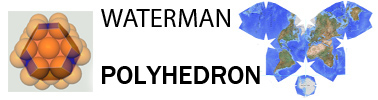challenge to currently applied mathematical procedure for Cartesian coordinate transformations wherein"any origin repositioning from initial coincindence, alters coordinations wrt their own repositioned system."Whereas, it is proposed that coordinates and selected points stay fixed wrt to their own system, during, or after any origin relocation; indeed, forever fixed in relationship to their own system.
 GIVEN SCENARIO / CONDITION MATH LOGIC coincident coordinate systems A with (x,y,z) and B with (x',y',z')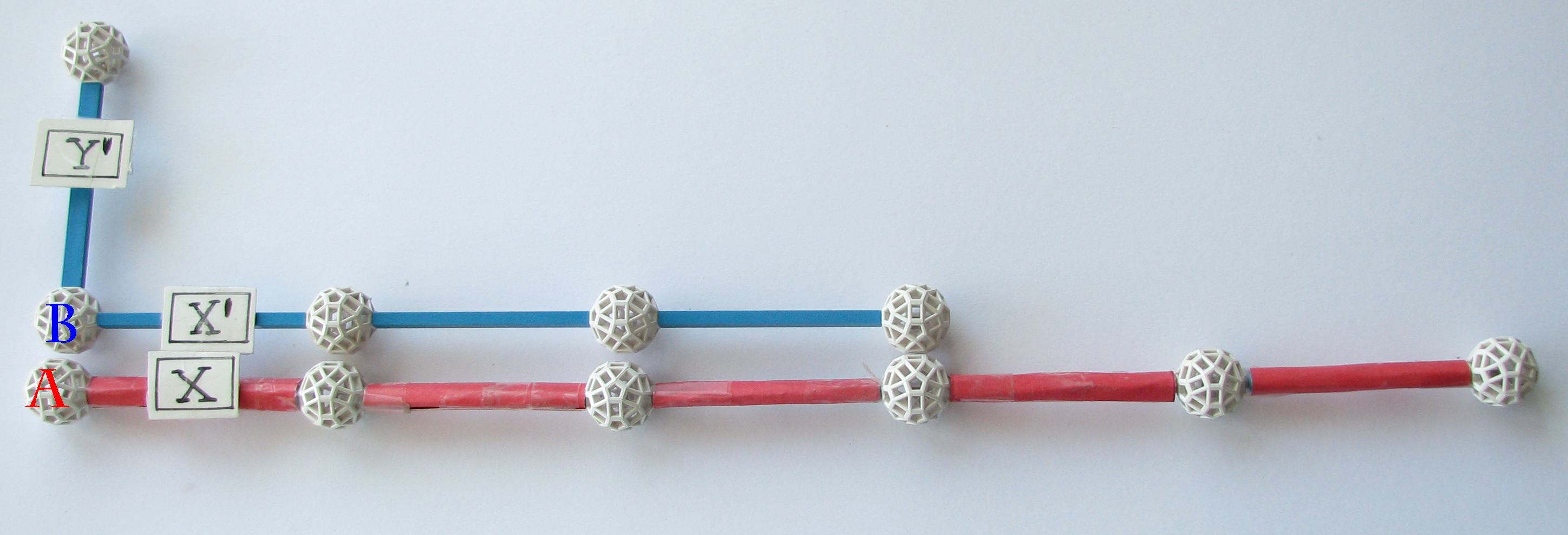1 A (x,y,z) = B (x',y',z') A was relocated left 3 or B was relocated right 3along x/x' direction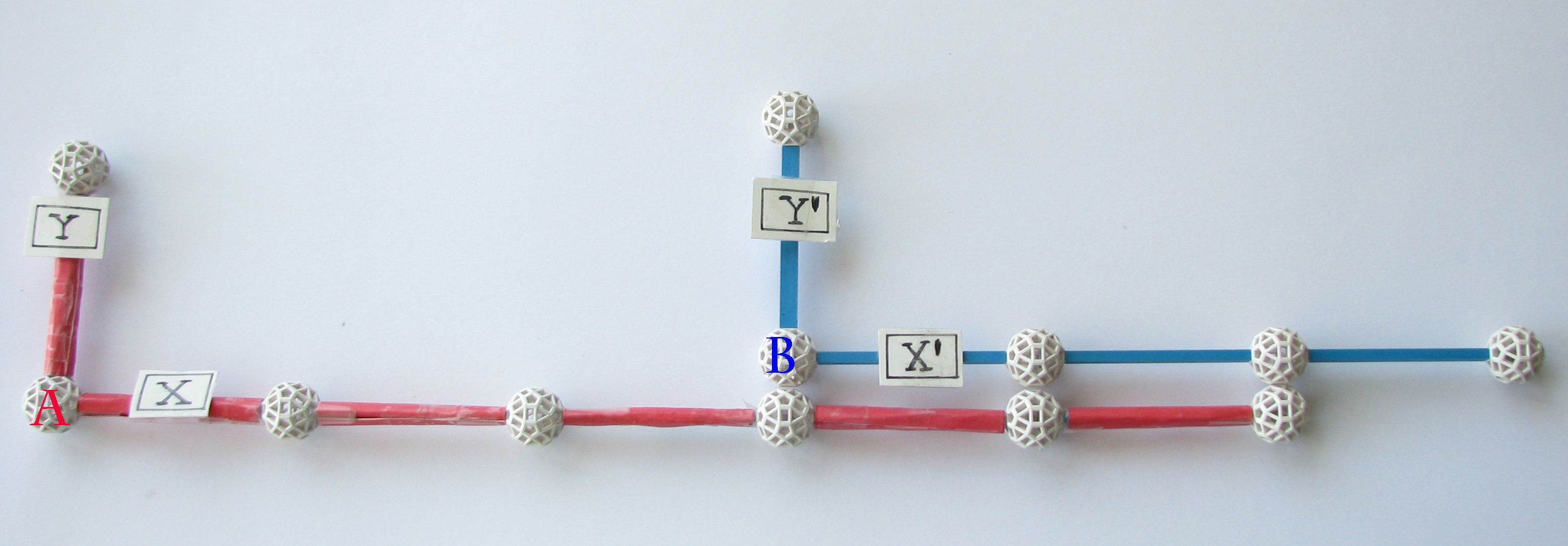2 It is impossible to know which has occurred from the depiction.A (x,y,z) = B (x',y',z') origin relocationrandom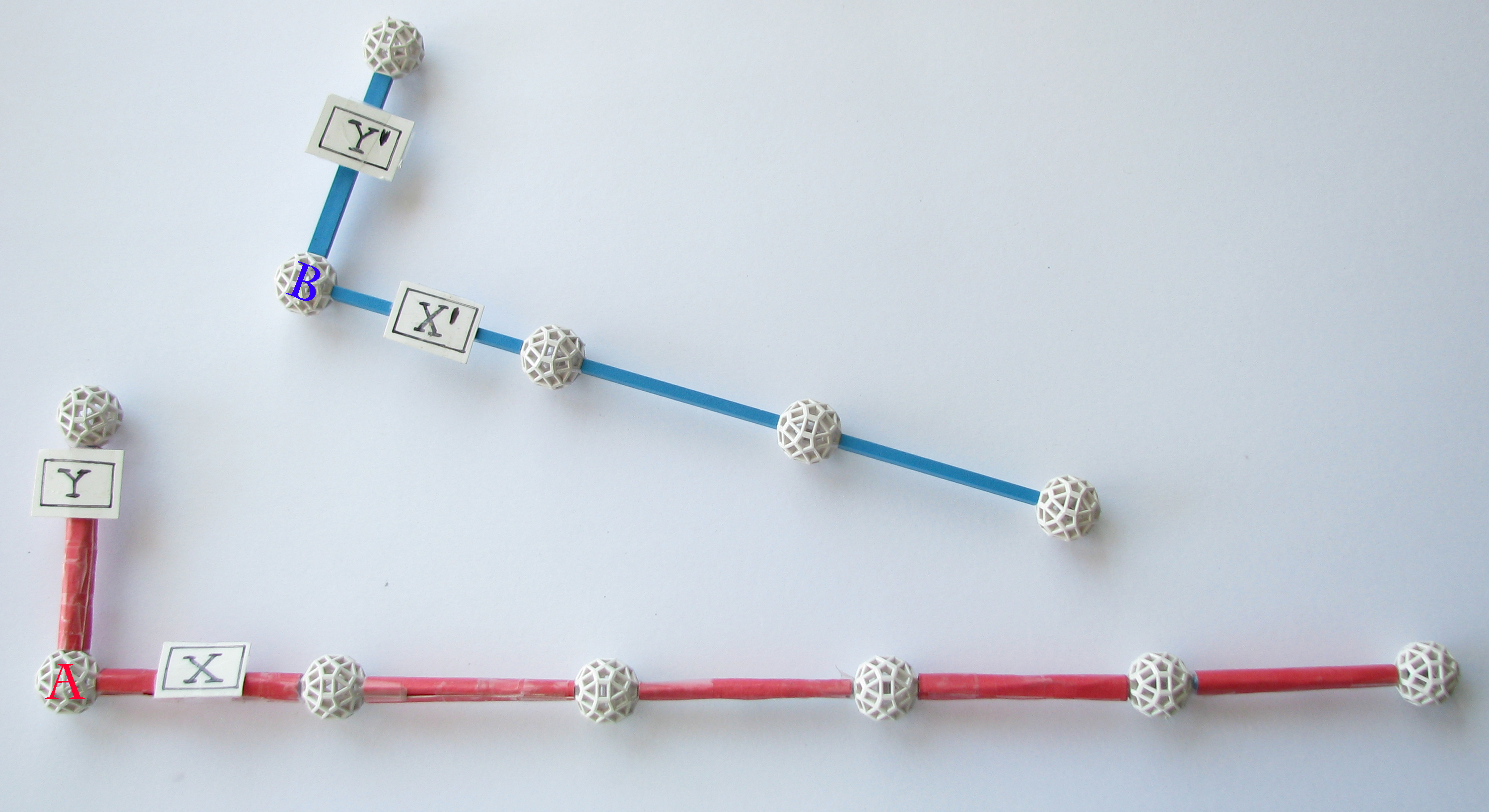3 A (x,y,z) = B (x',y',z') select point P in A = (2,0,0)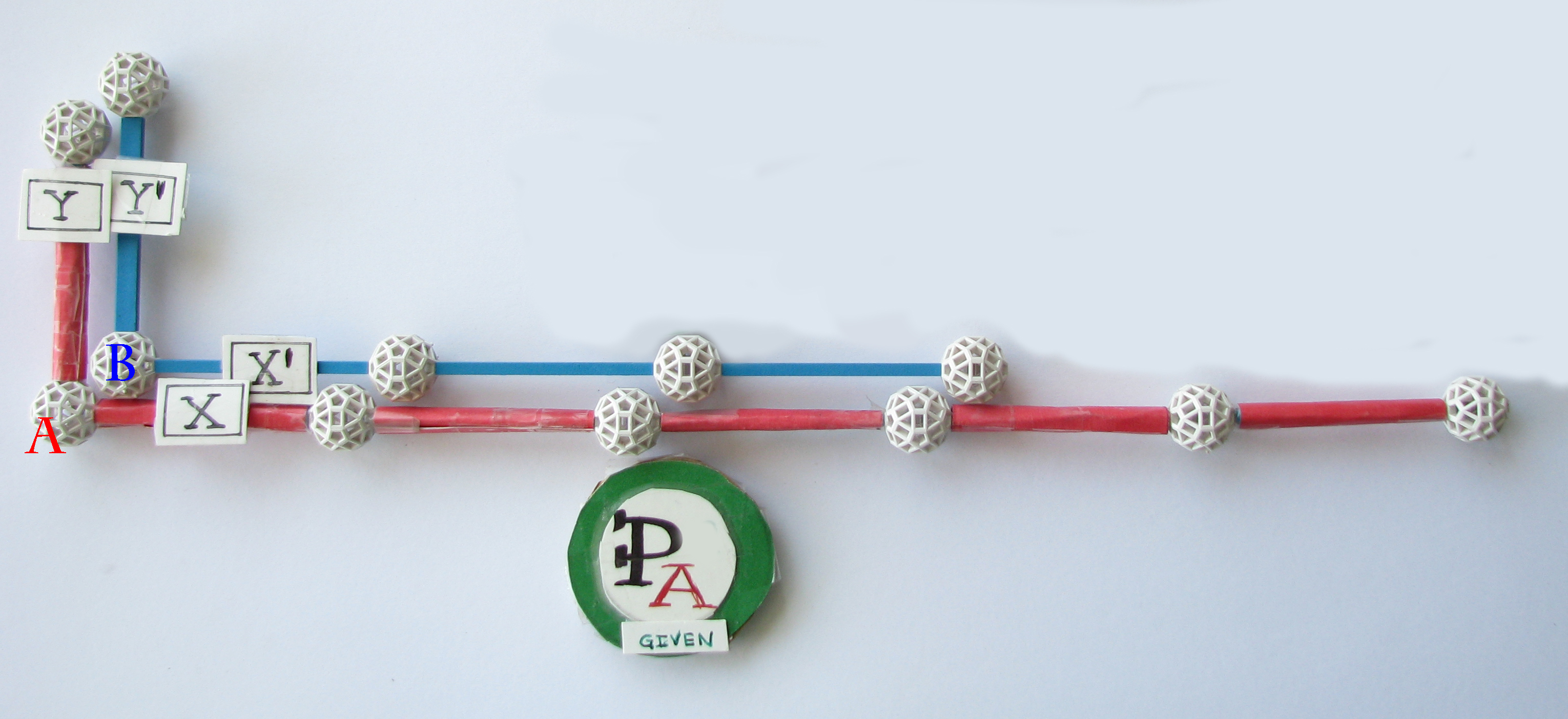4.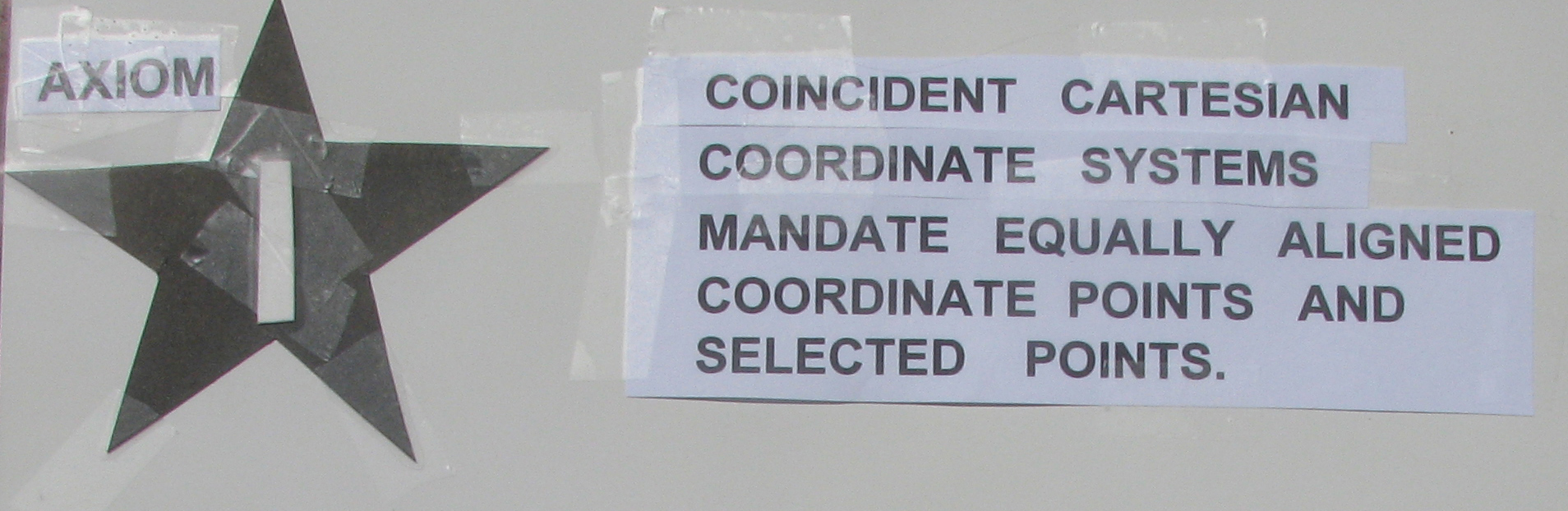select point P in A = (2,0,0)point P' in B = (2,0,0)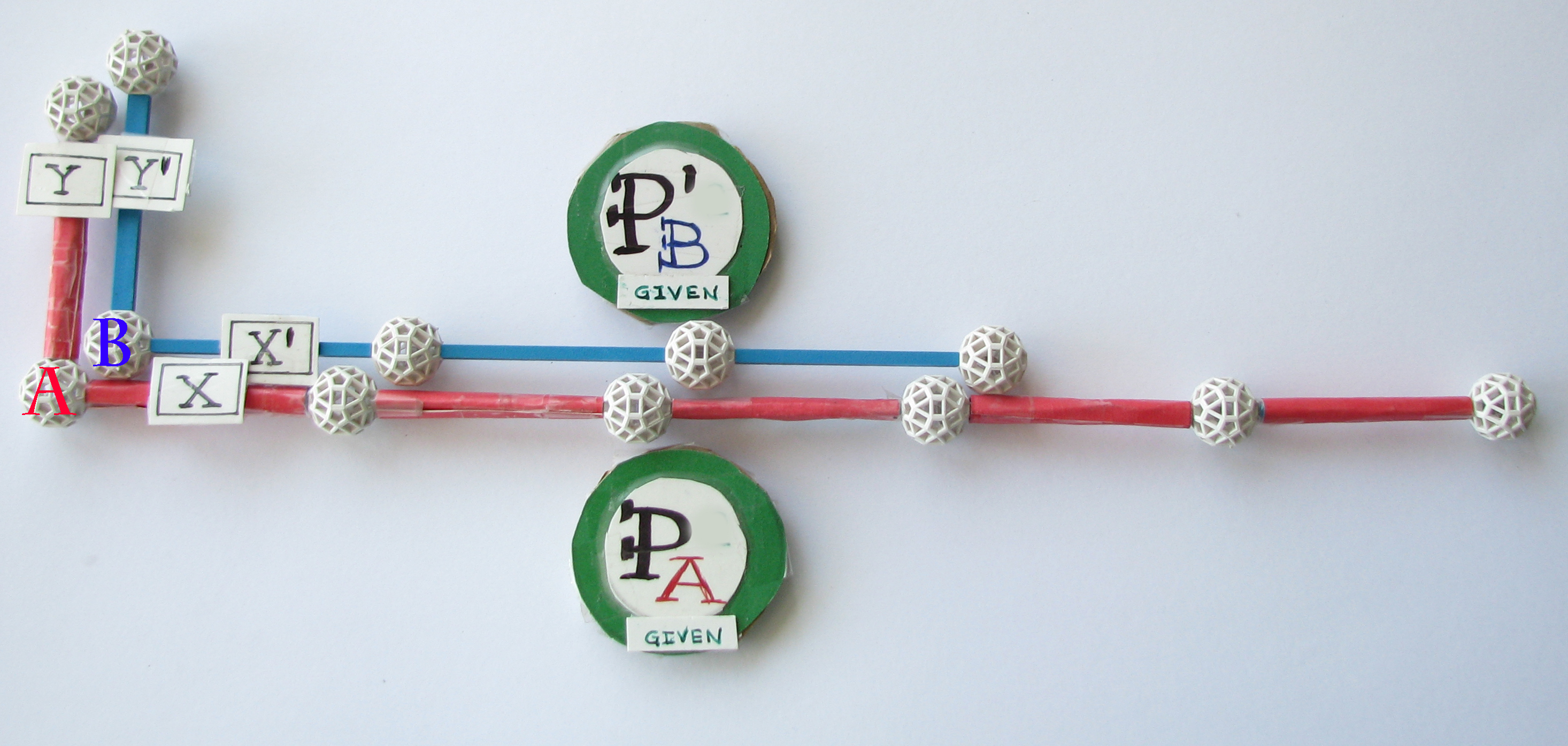5This is not a coordinate transformation between systems. method 1 origin relocation take diagram 5 and relocate B right 3 along the x/x' direction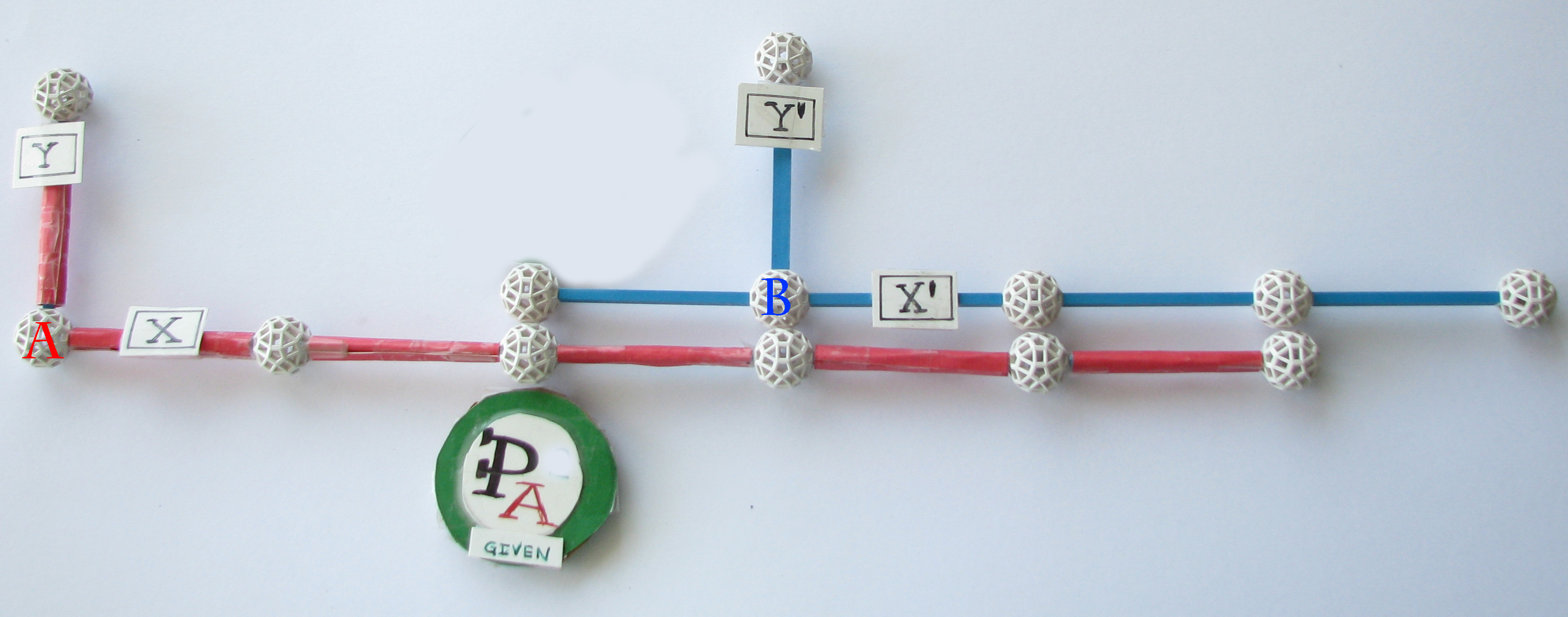6point P' in B is mysteriously missing?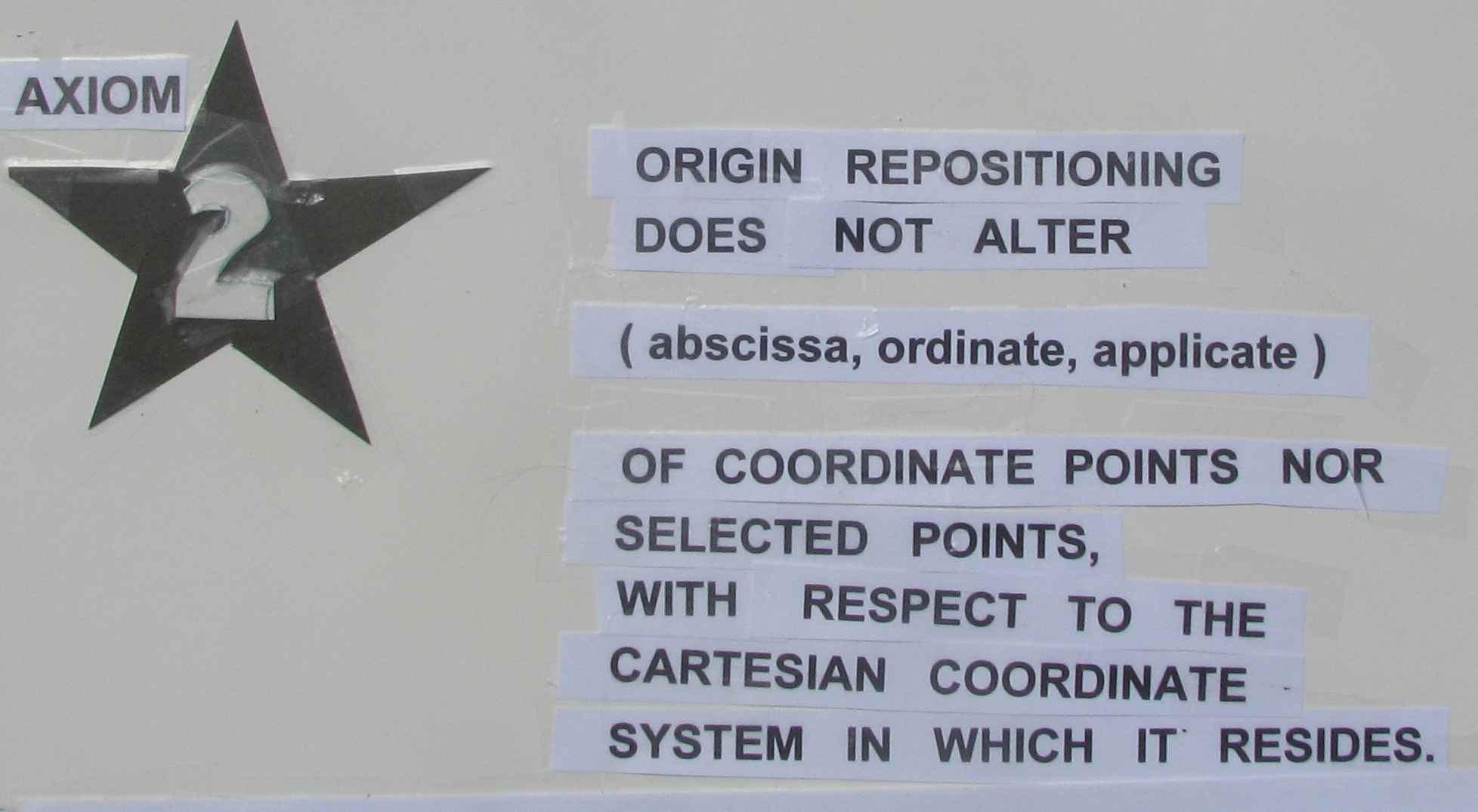method 2 origin relocation take diagram 5 and relocate B right 3 or A left 3 along the x/x' direction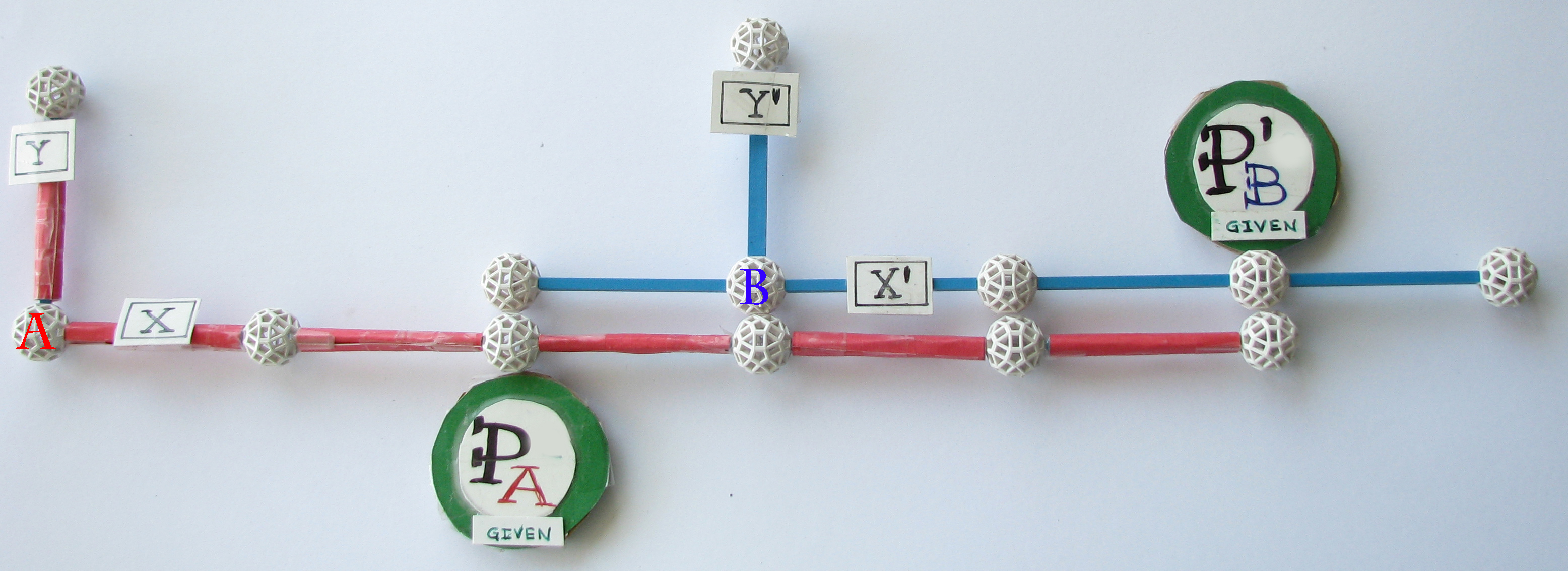7 This is not a coordinate transformation yet... not until an actual transfer between systems occurs. coordinate transformation related Physics terms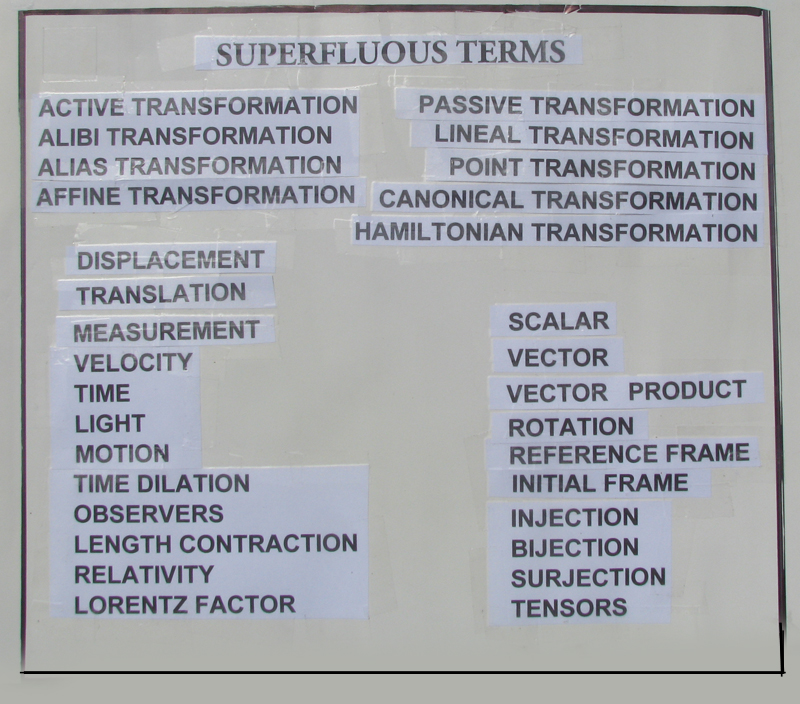8 These are not pertinent to a mathematical analysis given that Velocity x Time equals a purely mathematical relocation Distance.

The transformation of selected points between systems.

Givenrelocate A left 3 compared to relocate B right 3

 transformation method 1 [ ignores axiom 2 ] transformation method 2 [ applies axiom 2 ] variant transformations dependending upon which system is chosen as stationaryB relocated right 3...A stationarycommencing with depiction 6the transformation between systems is...invariant transformationindependent upon which system chosen as stationary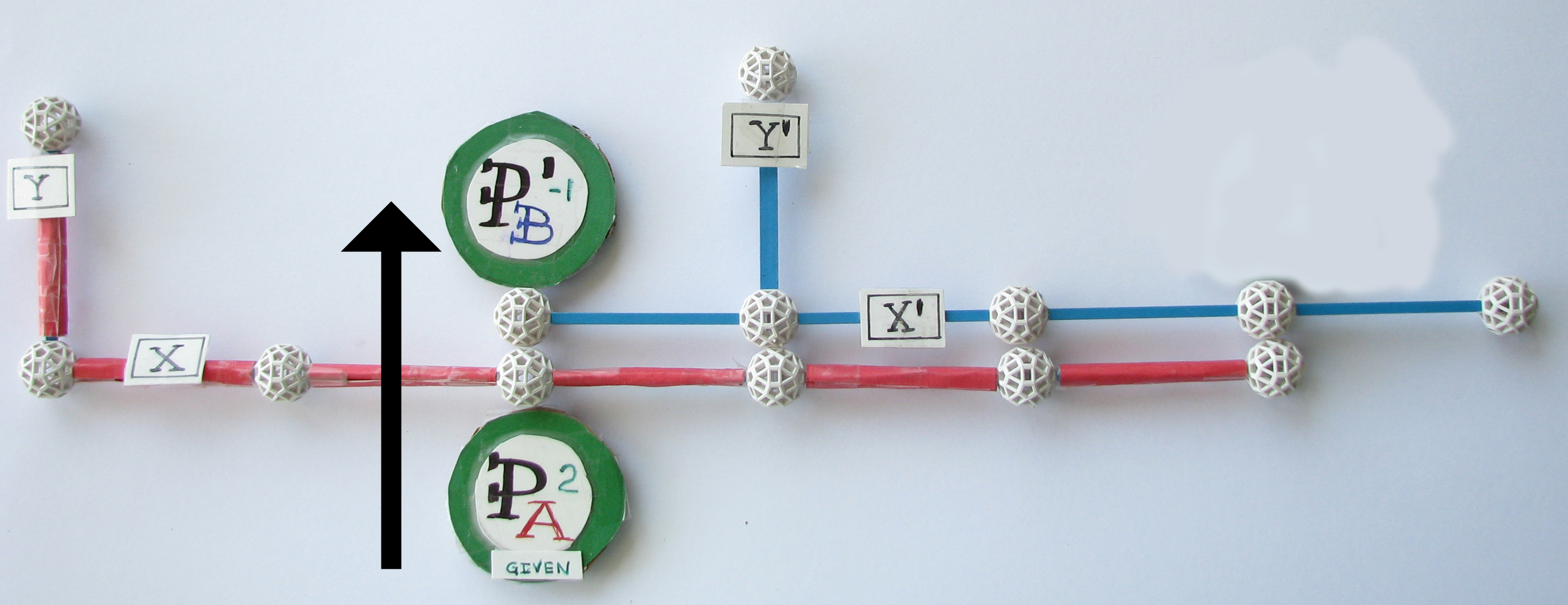B relocated right 3 or A left 3 commencing with depiction 7the transformation between systems is...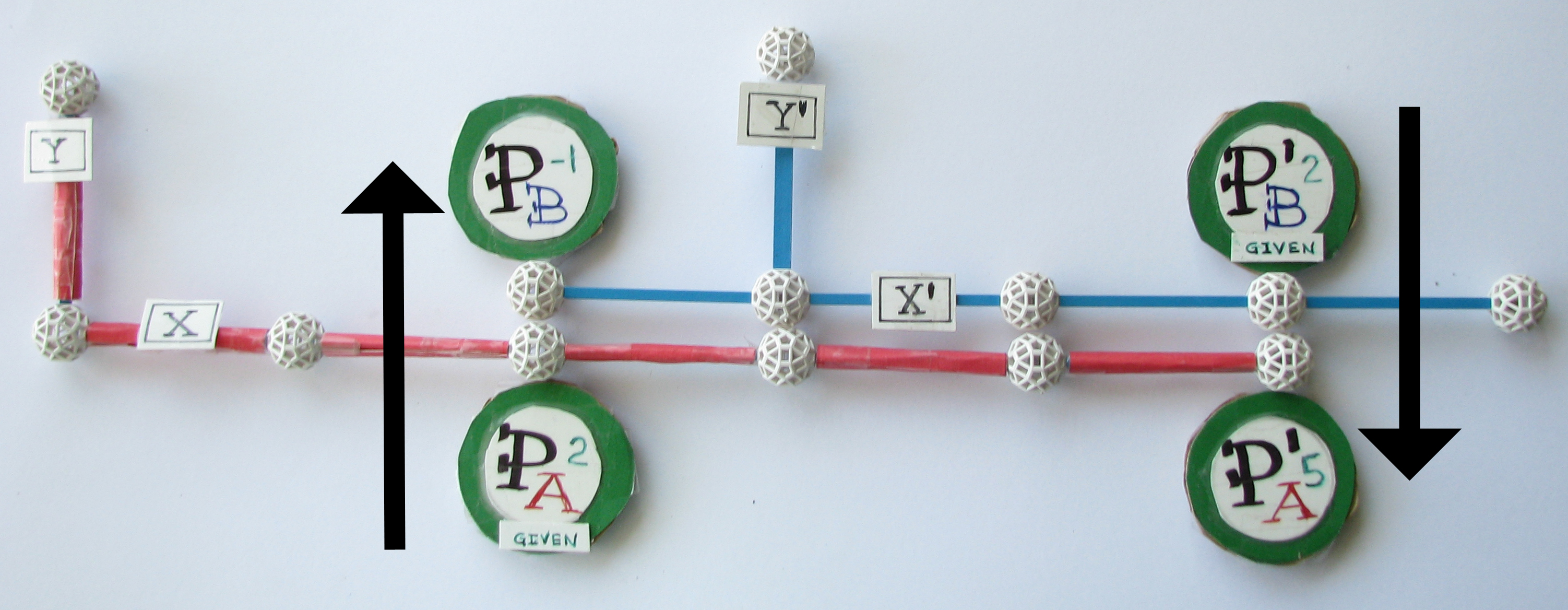A relocated left 3...B stationarythis time...it commences with the relocated singular point in the opposite system.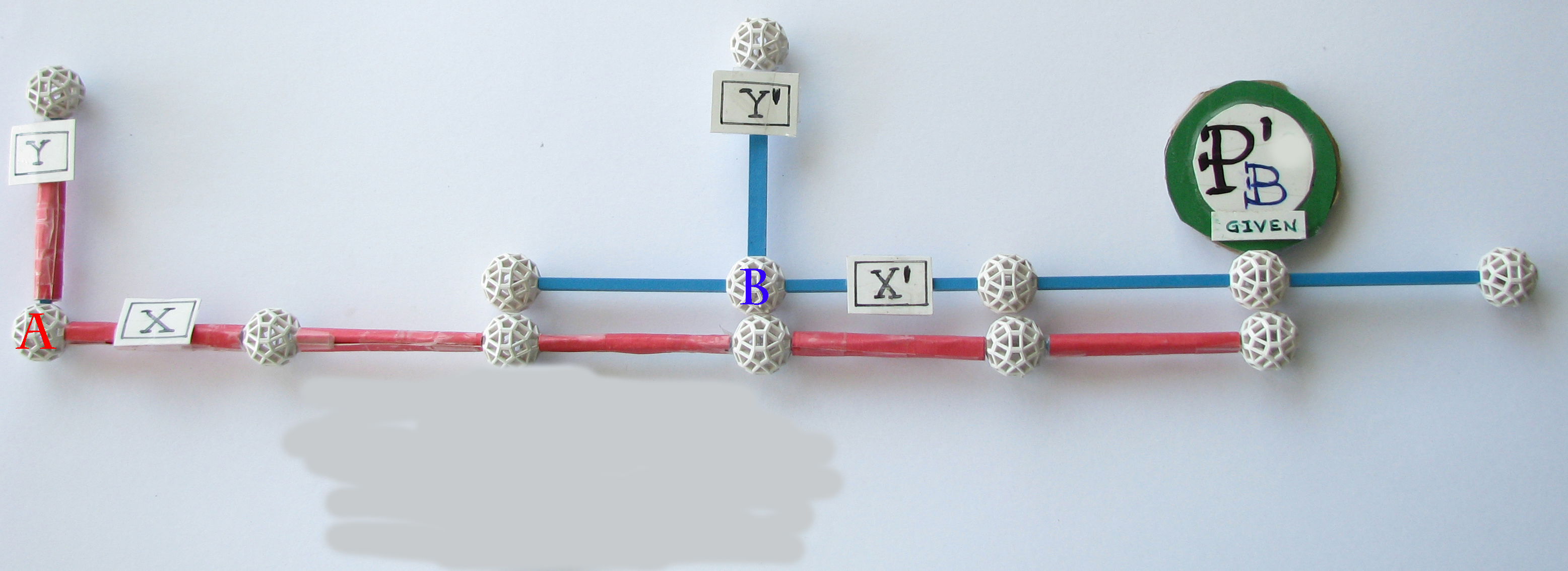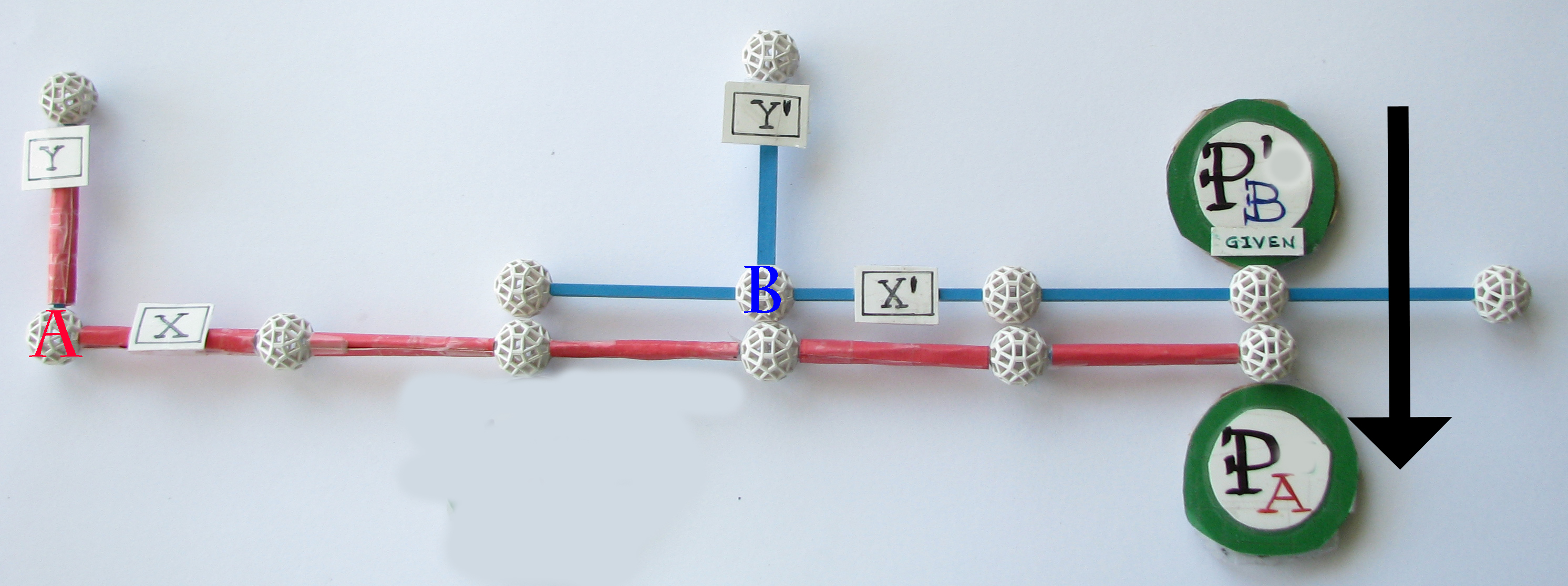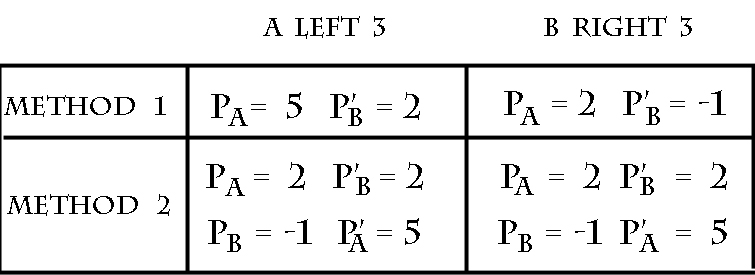conclusion

method 1 variant transformation results

The value of P in A is dependent upon which system was relocated
IF A left 3, THEN P in A = 5      IF B right 3, THEN = P in A = 2

The value of P' in B is dependent upon which system was relocated
IF A left 3 = THEN P' in B = 2      IF B right 3 = THEN P' in B = -1

method 2 invariant transformation results

coordinates and selected points stayed fixed wrt their origin, P in A = 2 P' in B = 2,
regardless of origin relocation.
As well,
P in A TRANSFOMS to P in B - ( relocated distance )
P' in B TRANFORMS to P' in A + ( relocated distance )

Conclusion Galilean A left 3 is variant because that result is different than B right 3 results.

additional original poems inspired by 3 xkcd comics....related directly to this particular math challenge